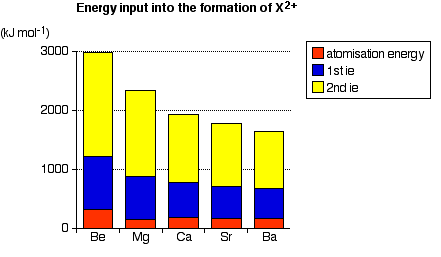# Reactions of Group 2 Elements with Water

•• Contributed by Jim Clark
• Former Head of Chemistry and Head of Science at Truro School in Cornwall

This page discusses the reactions of the Group 2 elements (beryllium, magnesium, calcium, strontium and barium) with water, using these reactions to describe the trend in reactivity in Group 2.

## Beryllium

Beryllium reacts with steam at high temperatures (typically around 700°C or more) to give white beryllium oxide and hydrogen.

$Be_{(s)} + H_2O_{(g)} \rightarrow BeO_{(s)} + H_{2(g)} \label{0}$

There is an additional reason for the lack of reactivity of beryllium compared with the rest of the Group. Beryllium has a strong resistant layer of oxide on its surface which lowers its reactivity at ordinary temperatures. However, the oxide layer breaks up above 750°C and exposes the beryllium metal surface below it, and so the protection then fails.

## Magnesium

Magnesium burns in steam to produce white magnesium oxide and hydrogen gas.

$Mg_{(s)} + H_2O_{(g)} \rightarrow MgO_{(s)} + H_{2(g)} \label{1}$

Very clean magnesium ribbon has a mild reaction with cold water, given below. After several minutes, hydrogen gas bubbles form on its surface, and the coil of magnesium ribbon usually floats to the surface. However, the reaction is short-lived because the magnesium hydroxide formed is almost insoluble in water and forms a barrier on the magnesium preventing further reaction.

$Mg_{(s)} + 2H_2O_{(l)} \rightarrow Mg(OH)_{2(s)} + H_{2(g)} \label{2}$

As a general rule, if a metal reacts with cold water, the metal hydroxide is produced. If it reacts with steam, the metal oxide is formed. This is because the metal hydroxides thermally decompose to the oxide and water.

## Calcium, Strontium, and Barium

These metals react with cold water with increasing vigor to give the metal hydroxide and hydrogen. Strontium and barium have reactivities similar to that of lithium. Calcium, for example, reacts fairly vigorously and exothermically with cold water. Bubbles of hydrogen gas are given off, and a white precipitate (of calcium hydroxide) is formed, together with an alkaline solution (also of calcium hydroxide, which is slightly water-soluble). The equation for the reactions of any of these metals would is as follows:

$X_{(s)} + 2H_2O_{(l)} + X(OH) \rightarrow X(OH)_{2 (aq\, or\, s)} + H_{2(g)} \label{3}$

The hydroxide solubilities increase down the group. Calcium hydroxide is mainly formed as a white precipitate (although some does dissolve). Less precipitate is formed down the group with increasing solubility.

## Thermodynamic Properties of the Reactions

The enthalpy change of a reaction is a measure of the amount of heat absorbed or evolved when the reaction takes place. An enthalpy change is negative if heat is evolved, and positive if it is absorbed. Calculate the enthalpy change for the possible reactions between beryllium or magnesium and steam gives the following values:

$Be_{(s)} + H_2O_{(g)} \rightarrow BeO_{(s)} + H_{2(g)} \;\;\; \Delta H = -369\; kJ/mol$

$Mg_{(s)} + H_2O_{(g)} \rightarrow MgO_{(s)} + H_{2(g)} \;\;\; \Delta H = -360\; kJ/mol$

Notice that both possible reactions are strongly exothermic, giving out almost identical amounts of heat. However, only the magnesium reaction actually happens. The explanation for the different reactivities must lie somewhere else. Similarly, calculating the enthalpy changes for the reactions between calcium, strontium or barium and cold water reveals that the amount of heat evolved in each case is almost exactly the same—about -430 kJ mol-1. The reason for the increase in reactivity must again lie elsewhere.

## Kinetics Properties of the Reactions

The activation energy for a reaction is the minimum amount of energy which is needed in order for the reaction to take place. It does not matter how exothermic the reaction would be once it got started - if there is a high activation energy barrier, the reaction will take place very slowly, if at all. When Group 2 metals react to form oxides or hydroxides, metal ions are formed. The formation of the ions from the original metal involves various stages all of which require the input of energy - contributing to the activation energy of the reaction. These stages involve the input of:

• the atomization energy of the metal. This is the energy needed to break the bonds holding the atoms together in the metallic lattice.
• the first + second ionization energies. These are necessary to convert the metal atoms into ions with a 2+ charge.

After this, there will be a number of steps which give out heat again - leading to the formation of the products, and overall exothermic reactions. The graph shows the effect of these important energy-absorbing stages as you go down Group 2.Notice that the ionization energies dominate this - particularly the second ionization energies. Ionization energies fall down the group. Because it gets easier to form the ions, the reactions will happen more quickly.

## Summarizing the Increase in Reactivity

The reactions of the Group 2 elements proceed more readily as the energy needed to form positive ions falls. This is mainly due to a decrease in ionization energy down the group. This leads to lower activation energies, and therefore faster reactions.